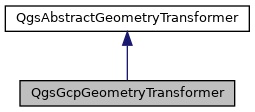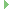QgsGcpGeometryTransformer Class Reference

A geometry transformer which uses an underlying Ground Control Points (GCP) based transformation to modify geometries. More...

`#include <qgsgcpgeometrytransformer.h>`

Inheritance diagram for QgsGcpGeometryTransformer:[legend]

## Public Member Functions

QgsGcpGeometryTransformer (const QgsGcpGeometryTransformer &other)=delete
QgsGcpGeometryTransformer cannot be copied. More...

QgsGcpGeometryTransformer (QgsGcpTransformerInterface *gcpTransformer)
Constructor for QgsGcpGeometryTransformer, which uses the specified gcpTransformer to modify geometries. More...

QgsGcpGeometryTransformer (QgsGcpTransformerInterface::TransformMethod method, const QVector< QgsPointXY > &sourceCoordinates, const QVector< QgsPointXY > &destinationCoordinates)
Constructor for QgsGcpGeometryTransformer, which uses the specified transform method and list of source and destination coordinates to transform geometries. More...

~QgsGcpGeometryTransformer () override

QgsGcpTransformerInterfacegcpTransformer () const
Returns the underlying GCP transformer used to transform geometries. More...

QgsGcpGeometryTransformeroperator= (const QgsGcpGeometryTransformer &other)=delete
QgsGcpGeometryTransformer cannot be copied. More...

void setGcpTransformer (QgsGcpTransformerInterface *transformer)
Sets the underlying GCP transformer used to transform geometries. More...

QgsGeometry transform (const QgsGeometry &geometry, bool &ok, QgsFeedback *feedback=nullptr)
Transforms the specified input geometry using the GCP based transform. More...

bool transformPoint (double &x, double &y, double &z, double &m) override
Transforms the point defined by the coordinates (x, y, z) and the specified m value. More...Public Member Functions inherited from QgsAbstractGeometryTransformer
virtual ~QgsAbstractGeometryTransformer ()=default

virtual bool transformPoint (double &x, double &y, double &z, double &m)=0
Transforms the point defined by the coordinates (x, y, z) and the specified m value. More...

## Detailed Description

A geometry transformer which uses an underlying Ground Control Points (GCP) based transformation to modify geometries.

Since
QGIS 3.18

Definition at line 35 of file qgsgcpgeometrytransformer.h.

## ◆ QgsGcpGeometryTransformer() [1/3]

 QgsGcpGeometryTransformer::QgsGcpGeometryTransformer ( QgsGcpTransformerInterface * gcpTransformer )

Constructor for QgsGcpGeometryTransformer, which uses the specified gcpTransformer to modify geometries.

Ownership of gcpTransformer is transferred to the geometry transformer.

Definition at line 21 of file qgsgcpgeometrytransformer.cpp.

## ◆ QgsGcpGeometryTransformer() [2/3]

 QgsGcpGeometryTransformer::QgsGcpGeometryTransformer ( QgsGcpTransformerInterface::TransformMethod method, const QVector< QgsPointXY > & sourceCoordinates, const QVector< QgsPointXY > & destinationCoordinates )

Constructor for QgsGcpGeometryTransformer, which uses the specified transform method and list of source and destination coordinates to transform geometries.

Definition at line 27 of file qgsgcpgeometrytransformer.cpp.

## ◆ ~QgsGcpGeometryTransformer()

 QgsGcpGeometryTransformer::~QgsGcpGeometryTransformer ( )
overridedefault

## ◆ QgsGcpGeometryTransformer() [3/3]

 QgsGcpGeometryTransformer::QgsGcpGeometryTransformer ( const QgsGcpGeometryTransformer & other )
delete

QgsGcpGeometryTransformer cannot be copied.

## ◆ gcpTransformer()

 QgsGcpTransformerInterface * QgsGcpGeometryTransformer::gcpTransformer ( ) const

Returns the underlying GCP transformer used to transform geometries.

setGcpTransformer()

Definition at line 59 of file qgsgcpgeometrytransformer.cpp.

## ◆ operator=()

 QgsGcpGeometryTransformer & QgsGcpGeometryTransformer::operator= ( const QgsGcpGeometryTransformer & other )
delete

QgsGcpGeometryTransformer cannot be copied.

## ◆ setGcpTransformer()

 void QgsGcpGeometryTransformer::setGcpTransformer ( QgsGcpTransformerInterface * transformer )

Sets the underlying GCP transformer used to transform geometries.

Ownership is transferred to this object.

gcpTransformer()

Definition at line 64 of file qgsgcpgeometrytransformer.cpp.

## ◆ transform()

 QgsGeometry QgsGcpGeometryTransformer::transform ( const QgsGeometry & geometry, bool & ok, QgsFeedback * feedback = `nullptr` )

Transforms the specified input geometry using the GCP based transform.

Parameters
 geometry Input geometry to transform ok will be set to `true` if geometry was successfully transformed, or `false` if an error occurred feedback This optional argument can be used to cancel the transformation before it completes. If this is done, the geometry will be left in a semi-transformed state.
Returns
transformed geometry

Definition at line 43 of file qgsgcpgeometrytransformer.cpp.

## ◆ transformPoint()

 bool QgsGcpGeometryTransformer::transformPoint ( double & x, double & y, double & z, double & m )
overridevirtual

Transforms the point defined by the coordinates (x, y, z) and the specified m value.

Parameters
 x point x coordinate y point y coordinate z point z coordinate, or NaN if the input point is 2D only m point m value, or NaN if not available
Returns
`true` if point was transformed (or no transformation was required), or `false` if point could not be transformed successfully.

### Example

A transformer which multiples the x coordinate by 3 and adds 10 to the y coordinate:

class MyTransformer(QgsAbstractGeometryTransformer):
def transformPoint(self, x, y, z, m):
# returns a tuple of True to indicate success, then the modified x/y/z/m values
return True, x*3, y+10, z, m
An abstract base class for classes which transform geometries by transforming input points to output ...

Implements QgsAbstractGeometryTransformer.

Definition at line 35 of file qgsgcpgeometrytransformer.cpp.

The documentation for this class was generated from the following files: